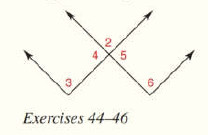Chapter 1.CR, Problem 45CR### Elementary Geometry for College St...

6th Edition
Daniel C. Alexander + 1 other
ISBN: 9781285195698

#### Solutions

Chapter
Section### Elementary Geometry for College St...

6th Edition
Daniel C. Alexander + 1 other
ISBN: 9781285195698
Textbook Problem
1 views

# Write two-column proofs for Review Exercises 39 to 46. Given: Figure as shown Prove: ∠ 4 is supp to ∠ 2 .To determine

To find:

The two-column proof for 4 is supplementary to 2.

Explanation

Given:

The given diagram is,

Figure (1)

The adjacent angles 4 and 2 are exterior sides of a straight line.

Definition:

If the sum of two angles is 180°, then the angles are known as supplementary angles. Each angle in the pair is the supplement of the other angle.

Approach:

The reasons for given statements is explained in table below.

 Statements Reasons 1. The adjacent angles ∠4 and ∠2 are exterior sides of a straight line. 1. Given 2. ∠4 is supplementary to ∠2. 2

### Still sussing out bartleby?

Check out a sample textbook solution.

See a sample solution

#### The Solution to Your Study Problems

Bartleby provides explanations to thousands of textbook problems written by our experts, many with advanced degrees!

Get Started